# Remainder

 1 What is Remainder? (with illustration) 2 Finding Remainders using Long Division (with illustration) 3 Representing Remainder 4 Remainder Calculator 5 Properties of Remainder 6 Tips and Tricks 7 Solved Examples on Remainder 8 Challenging Questions on Remainder 9 Practice Questions on Remainder 10 Maths Olympiad Sample Papers 11 Frequently Asked Questions (FAQs)

We at Cuemath believe that Math is a life skill. Our Math Experts focus on the “Why” behind the “What.” Students can explore from a huge range of interactive worksheets, visuals, simulations, practice tests, and more to understand a concept in depth.

Book a FREE trial class today! and experience Cuemath’s LIVE Online Class with your child.

## What is Remainder? (with illustration)

### Meaning of Remainder

Remainder, as its name suggests, is something that "remains" after completing a task.

Assume that you have 15 cookies that you want to share with 3 of your friends, Mary, David, and Jake.You want to share the cookies equally among your friends and yourself.

You will begin distributing them in the following way.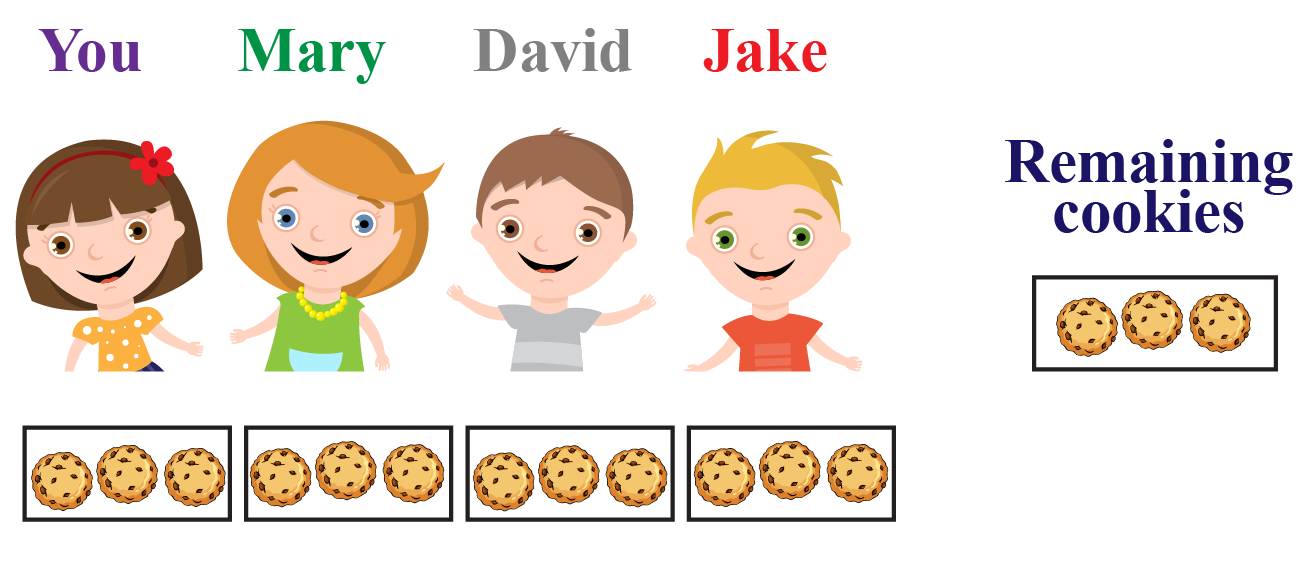Here, you can see that there are 3 cookies "remaining" after the distribution.

These 3 cookies cannot be further shared equally among the 4 of you.

Hence, 3 is called the "remainder" here.

We can understand the meaning of the remainder from the following illustration.

You can change the number of things and the number of groups by moving the corresponding sliders and see the remainder.

### Definition of Remainder

Remainder is a part of something that is "remaining" after division.

The remainder is left over when a few things are divided into groups with an equal number of things.

Let's recall the scenario we discussed earlier about 15 cookies being shared equally among $$4$$ children.

In other words, 15 cookies had to be divided into 4 equal groups.

We were left with 3 cookies and hence, 3 was the remainder.

Let us consider another example.

Let us assume that 8 slices of pizza are to be shared equally among 2 children.How many pieces of pizza remain unshared?

You can look at the picture below to understand how we have divided the slices of pizza equally between the two children.Thus, the remainder is 0 as no pieces of pizza are left unshared.

## Finding Remainders using Long Division (with illustration)

We cannot always pictorially show how we divide a number of things equally among the groups in order to find the remainder.

Instead, we can find the remainder using the long division method.

For example, the remainder in the above example on cookies can be found using long division as follows: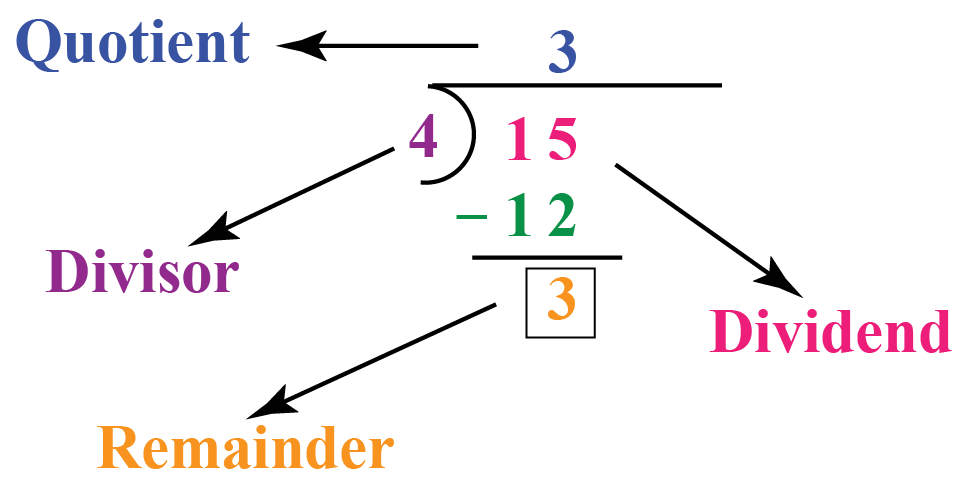The remainder of this division is 3.

The remainder in the above example on pizza can be found using long division as follows: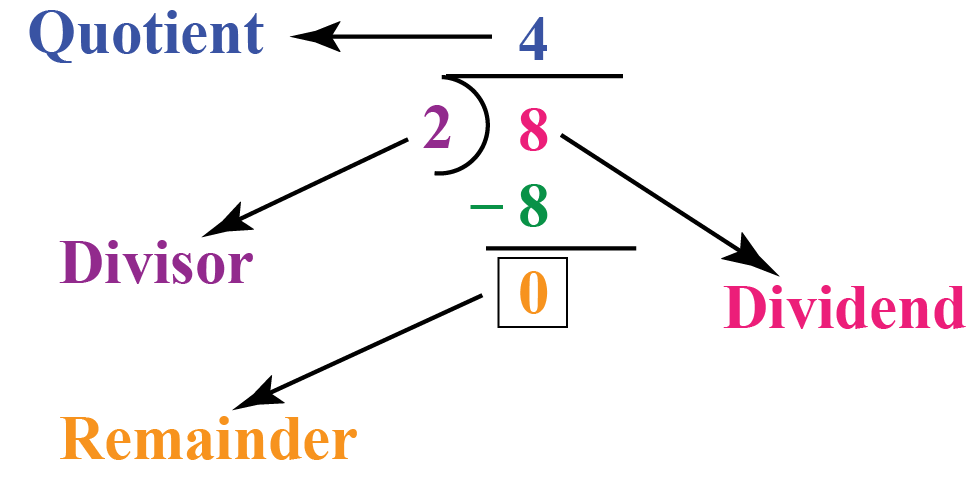The remainder of this division is 0.

### Examples of Remainder

Let us consider the division $$43 \div 2$$.

Let us find the remainder of this division using the following illustration.

You can use the "Play" and "Reset" buttons to view this illustration.

Thus, 1 is the remainder.

Here are some other examples of remainders.

Division

Remainder

$$35 \div 6$$

5

$$42 \div 8$$

2

$$121 \div 11$$

0

$$118 \div 12$$

10

$$120 \div 17$$

1

You can check these remainders by doing long division.

## Representing Remainder

Let us divide 7 by 2 using long division and see what the quotient and the remainder are.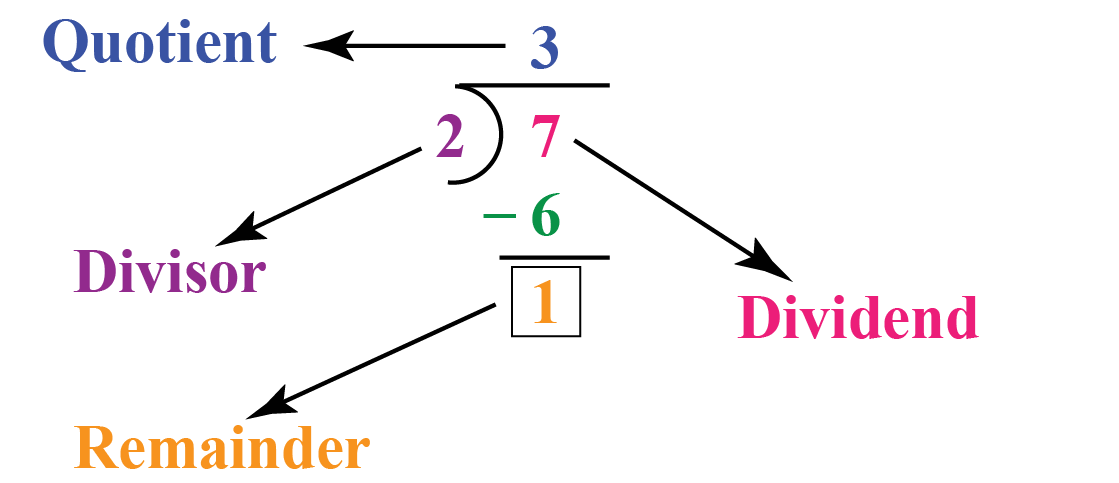We can represent the remainder of division in two ways.

One is by writing the quotient and the remainder with an "R" in between them.

 Dividend $$\div$$ Divisor = Quotient $$R$$ Remainder

For example, $$7 \div 2$$ can be written as:

$7 \div 2 = 3 \, R \, 1$

Another way to represent the remainder is by showing it as a part of a mixed fraction.

 $$\dfrac{ \text{Dividend}}{ \text{Divisor}} = \text{Quotient} \dfrac{ \text{Remainder}}{\text{Divisor}}$$

For example, $$7 \div 2$$ can be written as:

$7 \div 2 = 3 \dfrac{1}{2}$

## Remainder Calculator

We can enter the dividend and the divisor in the following calculator and it will show us the quotient and the remainder.

It also shows the division equation by clicking on the checkbox.

## Properties of Remainder

The properties of the remainder are as follows:

• The remainder is always less than the divisor. A remainder that is either greater than or equal to the divisor indicates that the division is incorrect.

• If one number (divisor) divides the other number (dividend) completely, then the remainder is 0.

• The remainder can be either greater, lesser, or equal to the quotient.Tips and Tricks
1. After solving a division problem, we can check the accuracy of the quotient and the remainder using the following equation.

Dividend = Divisor x Quotient + Remainder

We have to find the product of the "Divisor" and the "Quotient" and add this product to the "Remainder".
If this sum is the same as the "Dividend", the division you performed is correct.

Help your child score higher with Cuemath’s proprietary FREE Diagnostic Test. Get access to detailed reports, customised learning plans and a FREE counselling session. Attempt the test now.

Solved Examples

 Example 1

What is the remainder when 3723 is divided by 23?

Check if the answer you got is correct.

Solution:

Let us divide 3723 by 23 using long division.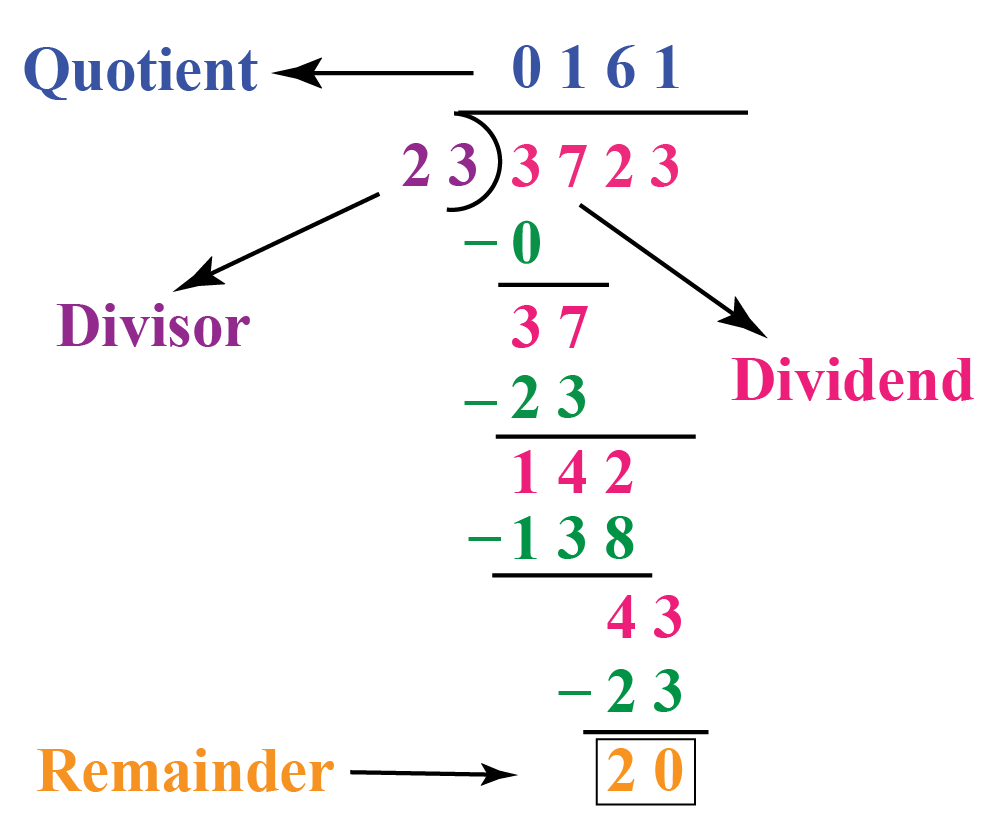Therefore,

 Remainder = 20

Now, we will check the answer by substituting all the values of dividend, divisor, quotient, and remainder in the following equation:

\begin{align}\text{Dividend }\!\!&= \!\!\text{ Divisor }\!\!\times\!\!\text{ Quotient }\!\!+\!\!\text{ Remainder}\\[0.2cm] 3723 &= 23 \times 161 + 20 \\[0.2cm] 3723 &= 3703+20\\[0.2cm] 3723&=3723 \end{align}

Since we have the same values on both the sides, our answer is correct.

 Example 2

The number of days in the year 1996 was 366 as it was a leap year.

If $$1$$st Jan 1996 was a Monday, what day was it on $$1$$st Jan 1997?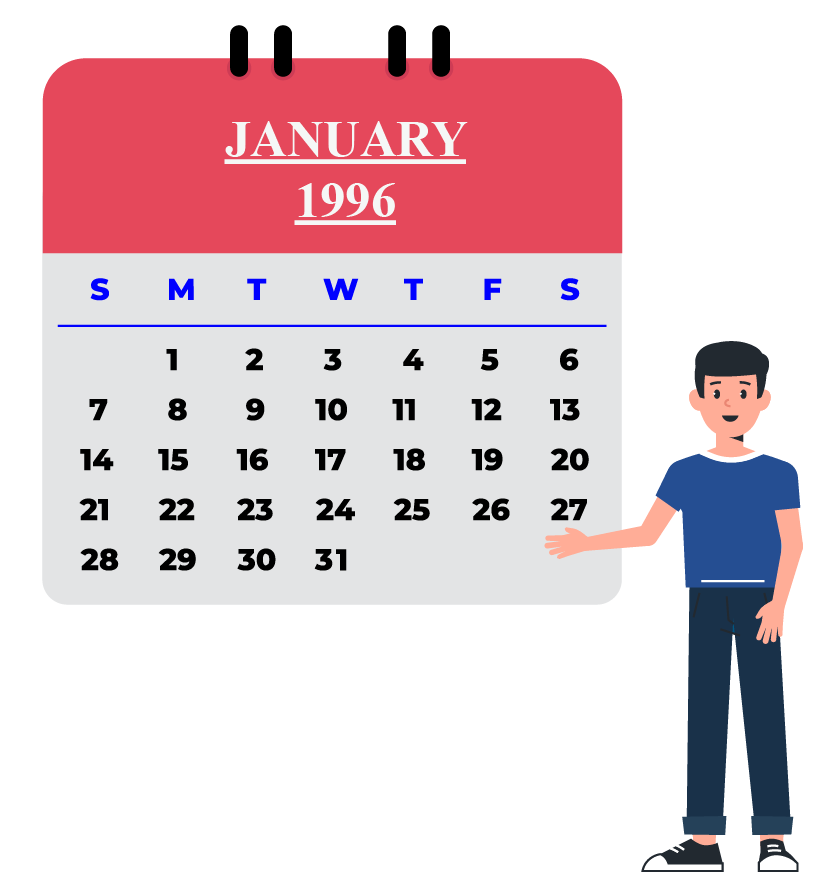Solution:

It is given that 1st Jan 1996 was a Monday.

We know that every day of the week repeats exactly after 7 days.

Hence, we will divide 366 by 7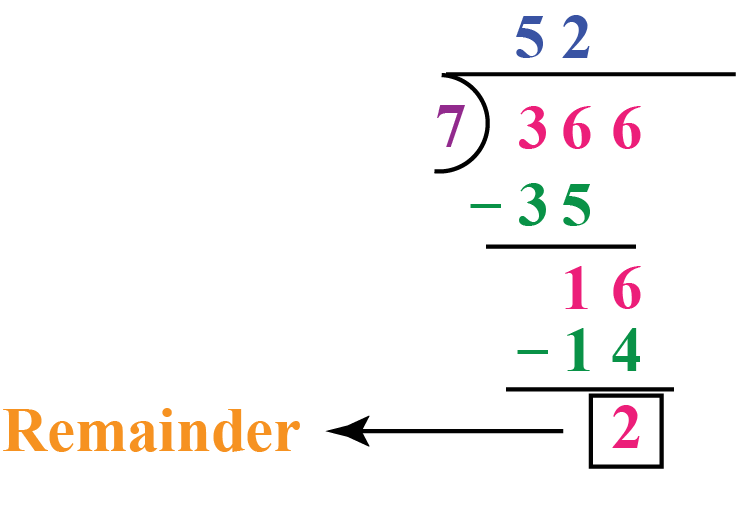2 is the remainder of division here.

We can say that 1st Jan 1997 = Monday + 2 days = Wednesday.

Hence,

 1st Jan 1997 was a Wednesday.
 Example 3

A company has a budget of Rs. 10,540 to buy external hard drives.

The cost of each hard drive is Rs. 450.

(i) How many hard drives can the company buy with the given budget?

(ii) How much money will the company be left with after the purchase?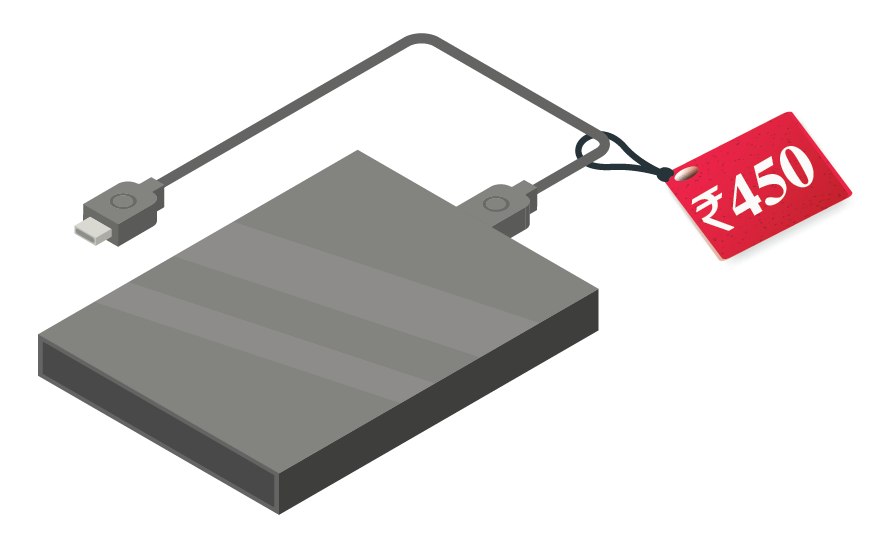Solution:

Total budget of the company = Rs. 10,540.

Cost of each hard drive = Rs. 450.

(i) We will divide 10,540 by 450 to find the number of hard drives that the company can buy.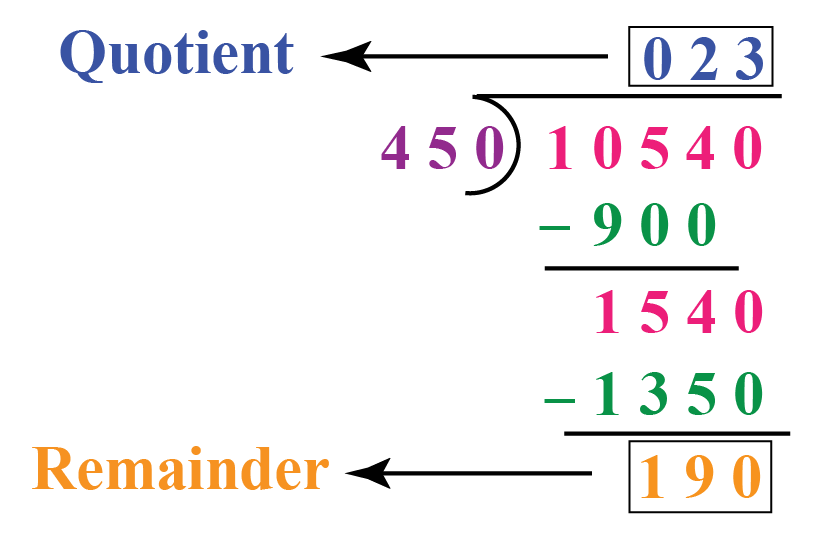The number of hard drives that the company can buy is nothing but the quotient of the division.

Therefore,

 Number of hard drives bought = 23

(ii) The amount of money that the company is left with is nothing but the remainder of the above division.

Therefore,

 Amount of money left = Rs. 190
 Example 4

A teacher distributes chocolates to 30 students in her class such that each one gets an equal number.If there are 315 chocolates in total,

(i) How many chocolates did each student get?

(ii) How many chocolates are left with the teacher after the distribution?

Solution:

Total number of students in the class = 30

Total number of chocolates that the teacher has = 315

(i) We will divide 315 by 30.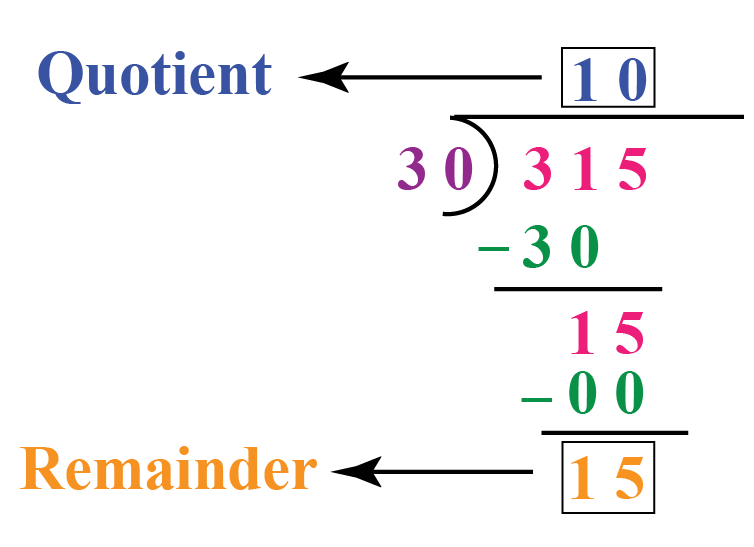The quotient of this division indicates the number of chocolates each student gets, which is 10.

Therefore,

 Chocolates each student gets = 10

(ii) The remainder of this division indicates the number of chocolates that are left with the teacher.

 Chocolates left with the teacher = 15Challenging Questions
1. John had 37 strawberries. If John gave equal numbers of strawberries to his 5 friends and then he ate what was left, how
many Starbursts did each person eat?
2. If Mary split 77 bananas between 7 people in her class and kept the leftovers for herself, how many bananas did each classmate ge

Have a doubt that you want to clear? Get it clarified with simple solutions on Remainder from our Math Experts at Cuemath’s LIVE, Personalised and Interactive Online Classes.

Make your kid a Math Expert, Book a FREE trial class today!

## Practice Questions

Here are a few activities for you to practice. Select/Type your answer and click the "Check Answer" button to see the result.

IMO (International Maths Olympiad) is a competitive exam in Mathematics conducted annually for school students. It encourages children to develop their math solving skills from a competition perspective.

## 1. What do you mean by remainder?

Remainder, as its name suggests, is something that "remains".

For more information, you can refer to the section, "What is remainder?"

## 2. What is an example of a remainder?

When 35 toffees are distributed equally among 8 children, each child gets 4 toffees and 3 toffees are left undistributed.

Here, 3 is the remainder.

You can find more examples of remainders under the following sections of this page.

"What is remainder?"

"Finding Remainders Using Long Division"

"Solved Examples"

## 3. How does a remainder work?

The definition of remainder is the part of a quantity that is left after dividing it into equal groups.

You can see how it works in real life using the "Solved Examples" and "Practice Questions" sections of this page.

## 4. Is zero a remainder?

Yes, 0 can be a remainder when the divisor divides the dividend completely.

For example, the remainder when 15 is divided by 3 is 0

More Important Topics
Numbers
Algebra
Geometry
Measurement
Money
Data
Trigonometry
Calculus